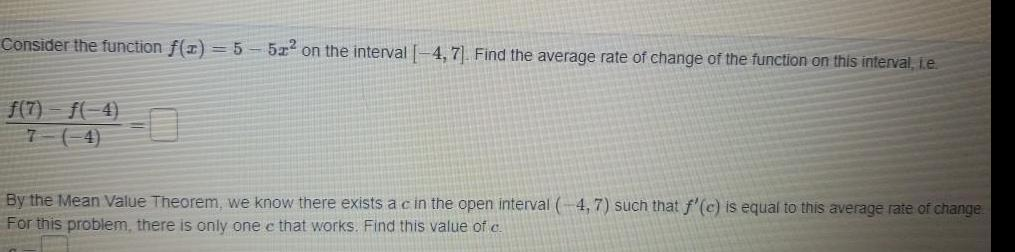Question:

# Consider the function f x 5 f 7 f 4 7 4 51 on the interval 4

Last updated: 9/19/2023Consider the function f x 5 f 7 f 4 7 4 51 on the interval 4 7 Find the average rate of change of the function on this interval i e By the Mean Value Theorem we know there exists a c in the open interval 4 7 such that f c is equal to this average rate of change For this problem there is only one e that works Find this value of c